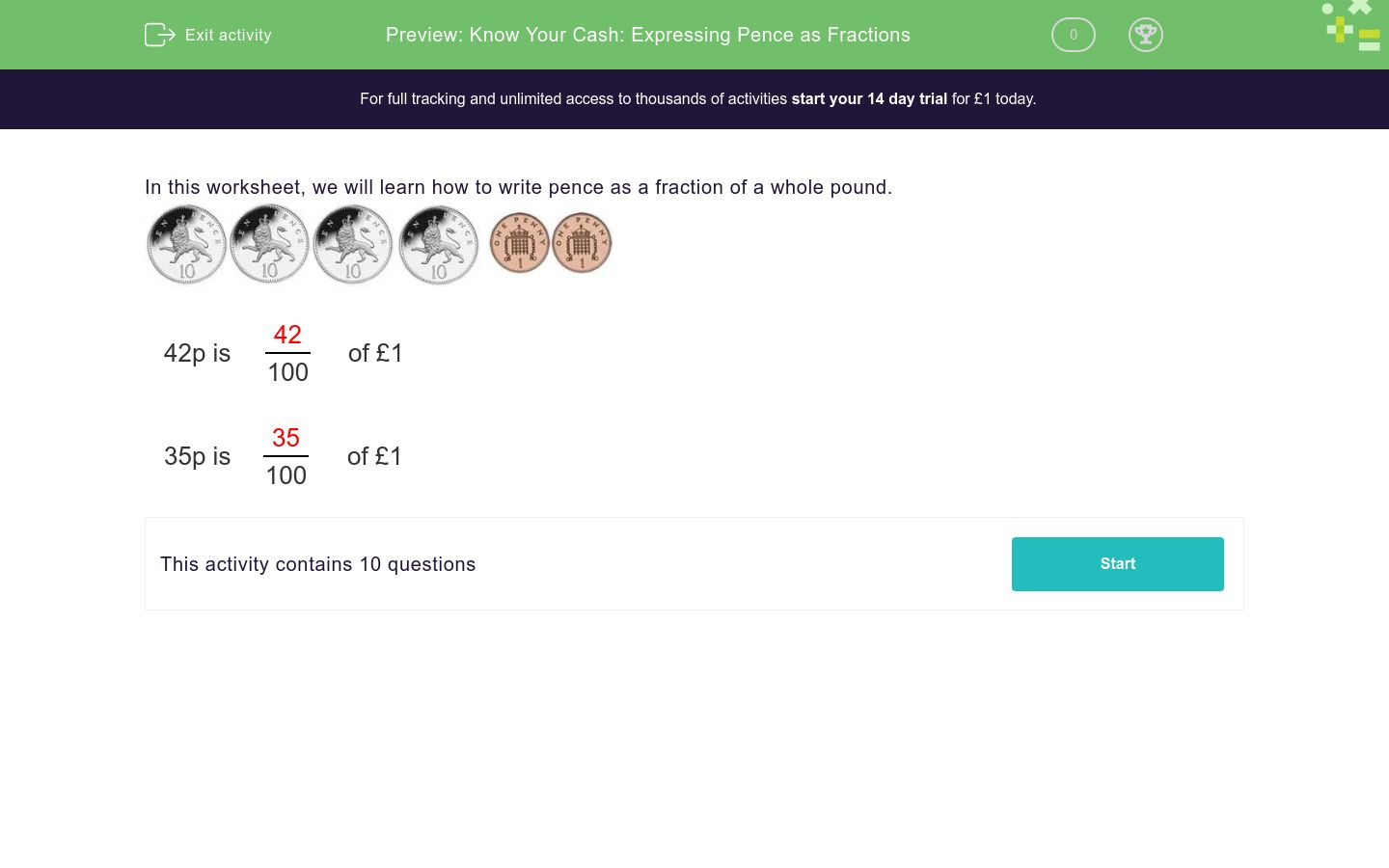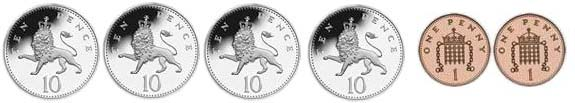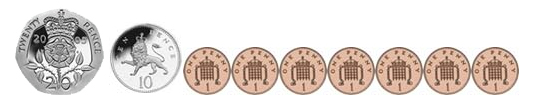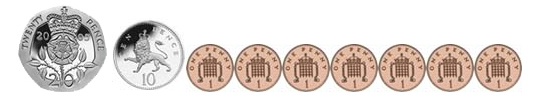# Know Your Cash: Expressing Pence as Fractions

In this worksheet, students convert a sum of money in pence to a fraction of a pound expressed in hundredths.Key stage:  KS 2

Curriculum topic:   Number: Fractions and Decimals

Curriculum subtopic:   Solve Fraction and Decimal Problems

Difficulty level:### QUESTION 1 of 10

In this worksheet, we will learn how to write pence as a fraction of a whole pound.42p is 42 of £1 100

 35p is 35 of £1 100Write as a fraction of £ 1

=
 100Write as a fraction of £ 1

=
 100Write as a fraction of £ 1

=
 100Write as a fraction of £ 1

=
 100Write as a fraction of £ 1

=
 100Write as a fraction of £ 1

=
 100Write as a fraction of £ 1

=
 100Write as a fraction of £ 1

=
 100Write as a fraction of £ 1

=
 100Write as a fraction of £ 1

=
 100

• Question 1Write as a fraction of £ 1

=
 100

18
• Question 2Write as a fraction of £ 1

=
 100

71
• Question 3Write as a fraction of £ 1

=
 100

37
• Question 4Write as a fraction of £ 1

=
 100

22
• Question 5Write as a fraction of £ 1

=
 100

76
• Question 6Write as a fraction of £ 1

=
 100

95
• Question 7Write as a fraction of £ 1

=
 100

38
• Question 8Write as a fraction of £ 1

=
 100

69
• Question 9Write as a fraction of £ 1

=
 100

37
• Question 10Write as a fraction of £ 1

=
 100

12
---- OR ----

Sign up for a £1 trial so you can track and measure your child's progress on this activity.

### What is EdPlace?

We're your National Curriculum aligned online education content provider helping each child succeed in English, maths and science from year 1 to GCSE. With an EdPlace account you’ll be able to track and measure progress, helping each child achieve their best. We build confidence and attainment by personalising each child’s learning at a level that suits them.

Get started